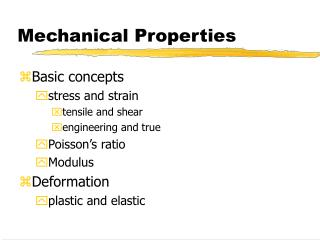# Mechanical Properties - PowerPoint PPT PresentationDownload PresentationMechanical Properties

Mechanical Properties
Download Presentation## Mechanical Properties

- - - - - - - - - - - - - - - - - - - - - - - - - - - E N D - - - - - - - - - - - - - - - - - - - - - - - - - - -
##### Presentation Transcript

1. Mechanical Properties • Basic concepts • stress and strain • tensile and shear • engineering and true • Poisson’s ratio • Modulus • Deformation • plastic and elastic

2. Mechanical Properties • Tensile properties • Elastic recovery • ductility

3. Tensile Stress and Strain • Stress • ratio of the perpindicular force applied to a specimen divided by its original cross sectional area. • Formally called engineering stress • Stress is measured in units of megapascals (MPa)

4. Tensile Stress and Strain • Strain • the ratio of change in length due to deformation to the original length of the specimen • formally called engineering strain • strain is unitless, but often units of m/m are used.

5. Tensile Stress and Strain

6. Shear Stress and Strain • Tensile stress is used in cases where purely sheer force is applied to a specimen and is given the symbol t. • Formula for calculation and units remain the same as tensile stress. • Differs from tensile stress only in the direction of the applied force (parallel for shear and perpendicular for tensile)

7. Shear Stress and Strain • Shear strain (g) is defined as the tangent of the angle q, and, in essence, determines to what extent the plane was displaced.

8. Stress-Strain Relationship • Hooke’s Law • for materials stressed in tension, at relatively low levels, stress and strain are proportional through: • constant E is known as the modulus of elasticity, or Young’s modulus. • Measured in MPa and can range in values from ~4.5x104 - 40x107 MPa

9. Stress-Strain Relationship • Shear stress and strain are related in a similar manner, but with a different constant of proportionality • the constant G is called the shear modulus and relates the shear stress and strain in the elastic region.

10. Poisson’s Ratio • When a material is placed under a tensile stress, an accompanying strain is created in the same direction. • As a result of this elongation, there will be constrictions in the other two directions. • Poisson’s ratio, n, is the ratio of the lateral to axial strains.

11. Poisson’s Ratio • Theoretically, isotropic materials will have a value for Poisson’s ratio of 0.25. • The maximum value of n is 0.5 • denotes no volume change during deformation. • Most metals exhibit values between 0.25 and 0.35 • It is also used to relate shear and elastic moduli

12. Plastic Deformation • Elastic deformation only occurs to strains of about 0.005. • After this point, plastic (non-recoverable) deformation occurs, and Hooke’s Law is no longer valid. • On an atomic level, plastic deformation is caused by slip, where atomic bonds are broken by dislocation motion, and new bonds are formed.

13. Plastic Deformation

14. Tensile Properties • Yield Strength • denoted sy, it is the strain corresponding to the elastic-plastic transition. • Calculated from a 0.002 strain offset from the origin, intersecting with the stress-strain curve. • Tensile Strength • stresses increase after yielding until a maximum is reached -- tensile strength (TS)

15. Tensile Properties • Prior to TS, the stress in the specimen is uniformly distributed. • After TS, necking occurs with localization of the deformation to the necking area, which will rapidly go to failure.

16. Elastic Recovery • After a load is released from a stress-strain test, some of the total deformation is recovered as elastic deformation. • During unloading, the curve traces a nearly identical straight line path from the unloading point • parallel to the initial elastic portion of the curve • The recovered strain is calculated as the strain at unloading minus the strain after the load is totally released.

17. Elastic Recovery

18. Ductility • Ductility is a measure of the degree of plastic deformation at fracture • expressed as percent elongation • also expressed as percent area reduction • lf and Af are length and area at fracture